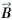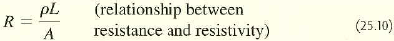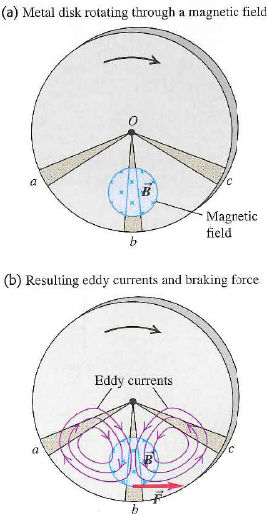Problem

# Consider a uniform metal disk rotating through a perpendicular magnetic field , as shown i...

Consider a uniform metal disk rotating through a perpendicular magnetic field, as shown in Fig. 29.19a. The disk has mass m, radius R, and thickness t, is made of a material with resistivity ρ, and is rotating clockwise in Fig. 29.19a with angular speed ω. The magnetic field is directed into the plane of the disk. Suppose that the region to which the magnetic field is confined is not circular, as shown in Fig. 29.19a, but is a small square with sides of length L (LR) centered a distance d from the point O (the center of the disk). The sides of this square are horizontal and vertical in Fig. 29.19a. (a) Show that the current induced within the square is approximately equal to I = ωdBLt/ρ. In which direction does this current flow? (Hint: Assume that the resistance to the current is confined to the region of the square. The current also encounters resistance as it flows outside the region to which the magnetic field is confined, as shown in Fig. 29.19b; however, this resistance is relatively small, since the current can flow through such a wide area. Recall Eq. (25.10) for resistance, given in Section 25.3.) (b) Show that the induced current gives rise to a torque of approximate magnitude τ = ωd2B2L2t/ρ that opposes the rotation of the disk (that is, a counterclockwise torque). (c) What would be the magnitudes and directions of the induced current and torque if the direction ofwere still into the plane of the disk but the disk rotated counterclockwise? What if the direction ofwere out of the plane and the disk rotated counterclockwise?Figure. 29.19 Eddy currents induced in a rotating metal disk.#### Step-by-Step Solution

Request Professional Solution

#### Request solution!

We need 10 more requests to produce this problem solution. Share with your friends to get the problem solution faster!

0 /10 have requested this problem solution

Once 10 people have made a request, the problem solutions will be available in 1-2 days.
All students who have requested the problem solution will be notified once they are available.